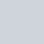# Probability Distributions in Statistics

In statistics, probability distributions are very important measure which related to the random variable and statistical data. Every data patern follows a probability distribution. Continuous data follow the continuous distribution and discrete data follow the discrete distributions. According to wikipedia,

“In probability theory and statistics, a probability distribution is the mathematical function that gives the probabilities of occurrence of different possible outcomes for an experiment. It is a mathematical description of a random phenomenon in terms of its sample space and the probabilities of events.”

### Probability distributions

The idea of a probability distribution exactly parallels that of a frequency distribution. Each type of distribution is based on a set of mutually exclusive and exhaustive measurement classes or class intervals. A probability distribution is thus an idealization of the way things might be if we only had all the information. It dictates what we should expect to observe in a frequency distributions, if some given state of affairs is true. Thus, we can say,

Any statement of a function associating each of a set of mutually exclusive and exhaustive classes or class intervals with its probability is called probability distribution.

A probability distribution is divided into two category. They are-

• Discrete probability distribution
• Continuous probability distribution

### Discrete Probability Distributions

A discrete random variable assumes each of its values or numbers with a certain probability. A probability distribution with discrete random variable is called discrete probability distribution.

There are some discrete probability distributions which are very important part of statistics are following-

### Continuous Distributions

When a probability distribution contains conrinuous random variable then the distribution is called continuous probability distribution.
Some impotant continuous probability distributions are following-

### One thought on “Probability Distributions in Statistics”

1.Hairstyles

Thanks for your article. I would love to remark that the first thing you will need to conduct is check if you really need repairing credit. To do that you will need to get your hands on a replica of your credit file. That should not be difficult, since the government necessitates that you are allowed to acquire one no cost copy of your credit report on a yearly basis. You just have to request that from the right people. You can either read the website for your Federal Trade Commission or even contact one of the major credit agencies right away.